>

# NCERT Solutions for Class 11 Maths Chapter 5 Complex Numbers and Quadratic Equations

In this page, you will get NCERT Solutions for Class 11 Maths Chapter 5 Complex Numbers and Quadratic Equations that can be used in solving difficult problems in the chapter. These NCERT Solutions for Class 11 will help you in grasping important points inside the chapter properly. You can download our Studyrankers app from Playstore to access NCERT Solutions for Class 11 Maths offline.

In Chapter 5 Complex Numbers and Quadratic Equations Class 11 Mathematics NCERT Solutions, you need to express given complex number in the form a + ib and multiplicative inverse of given complex numbers.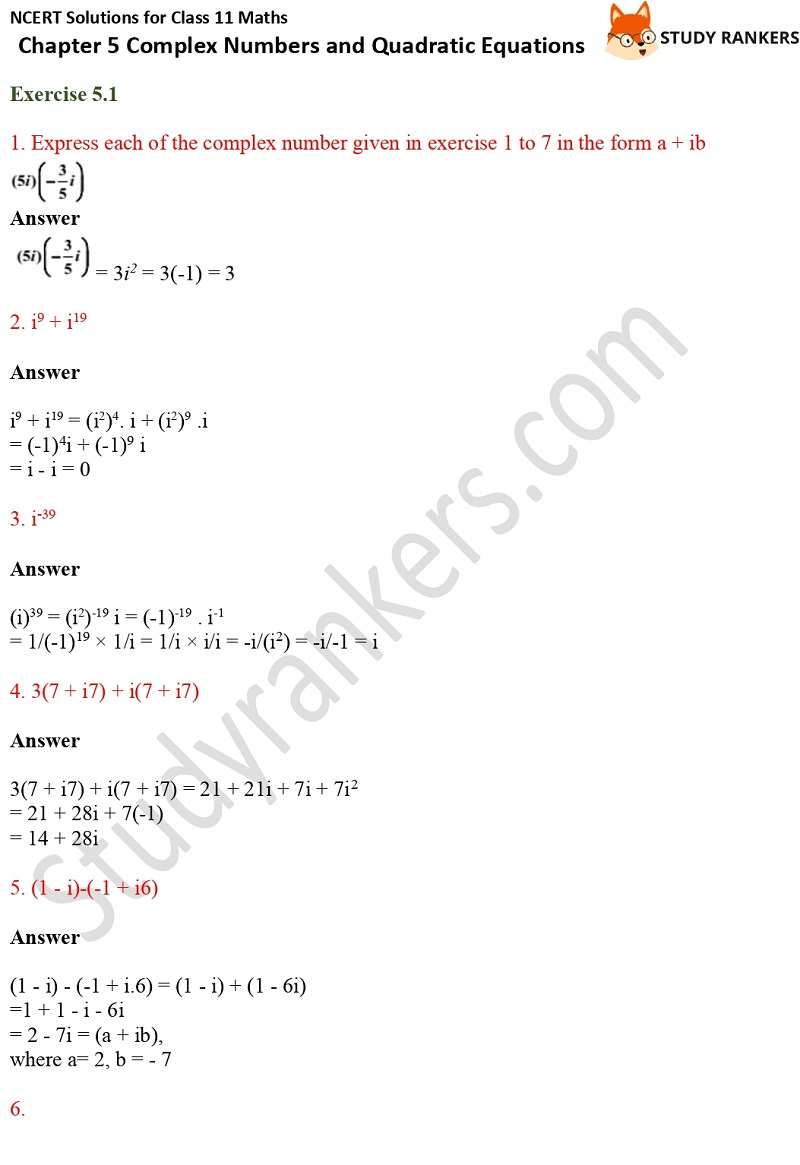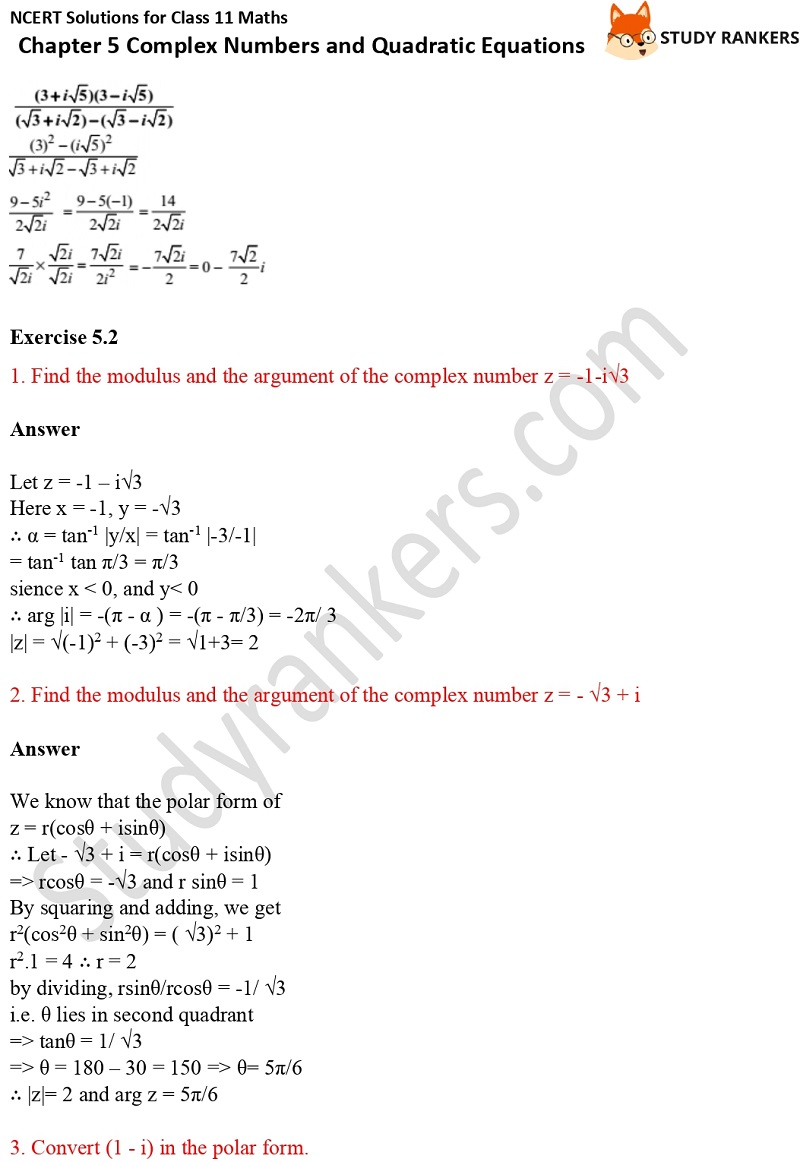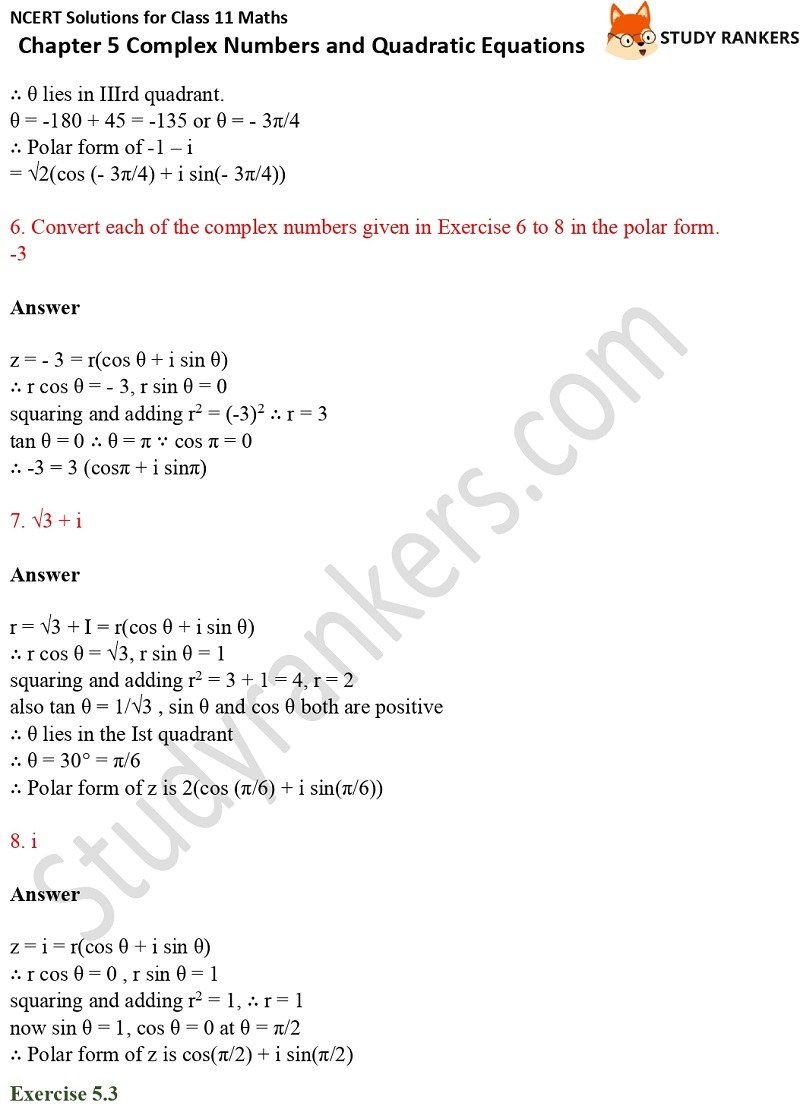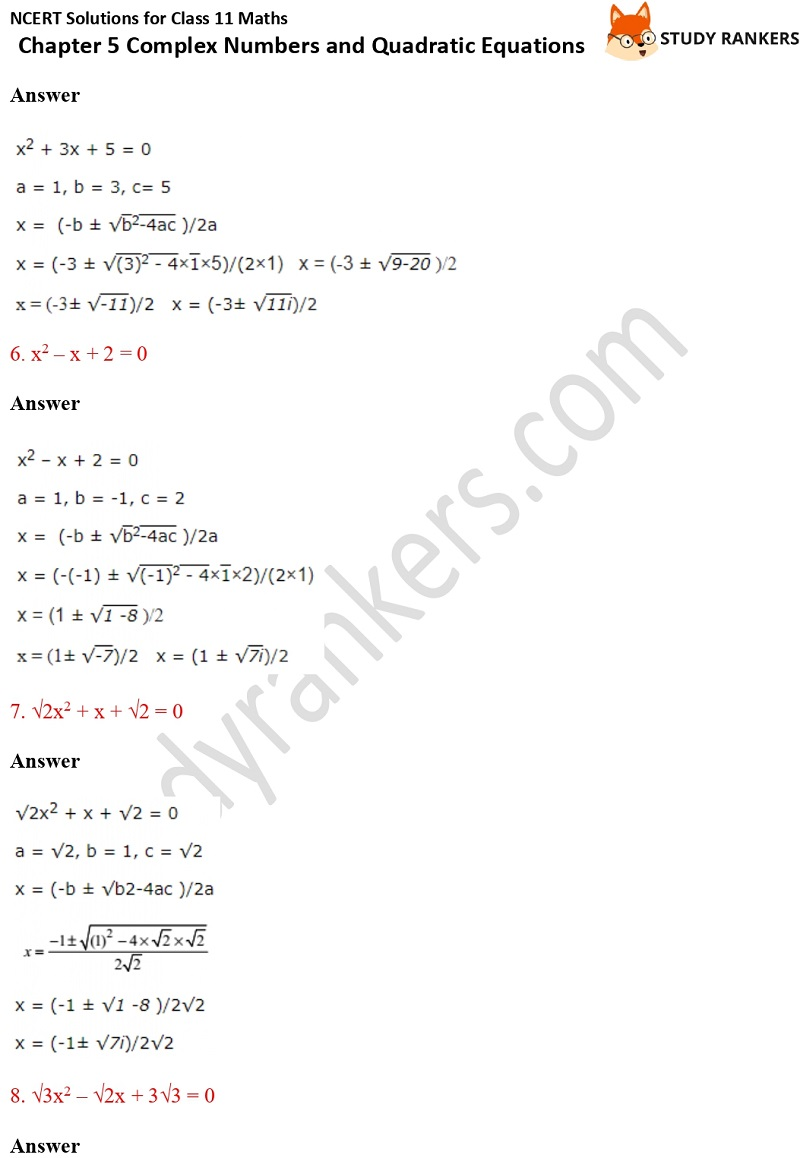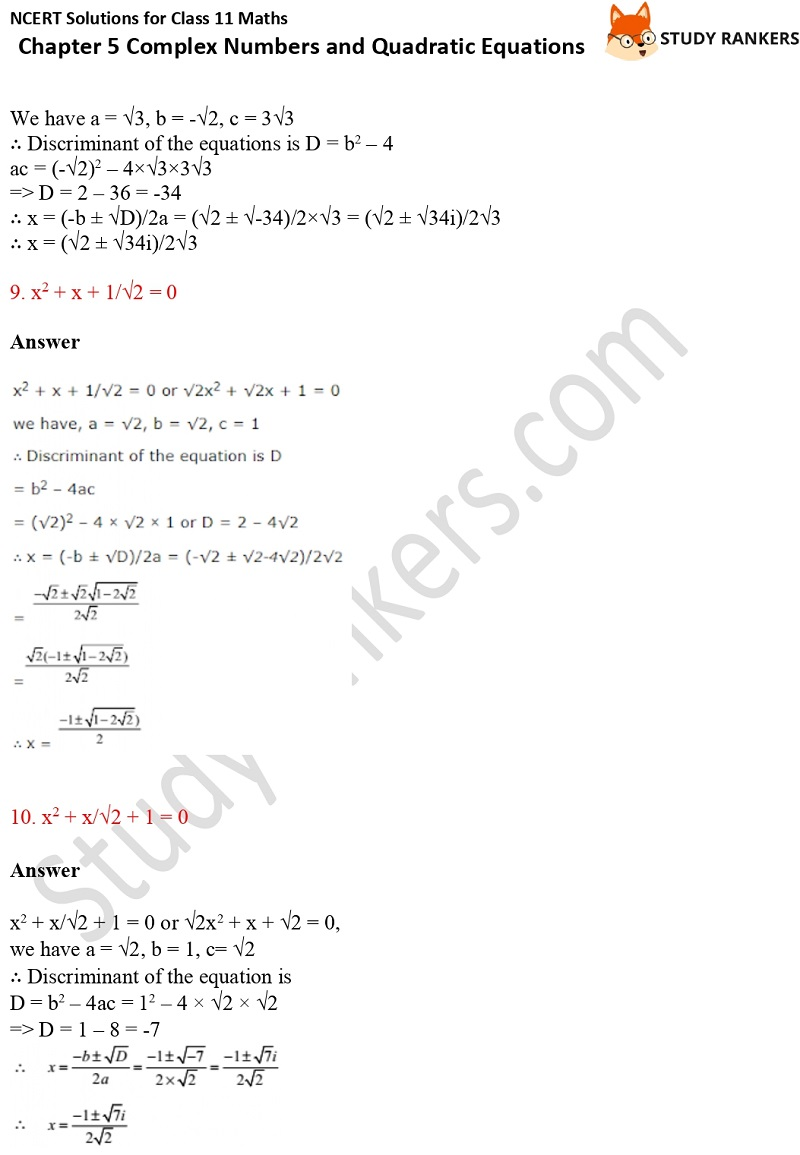X